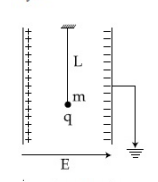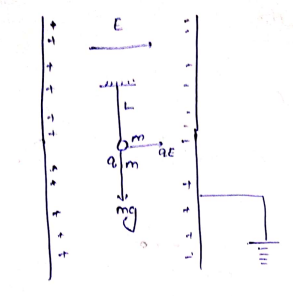Q

# Need clarity, kindly explain! A simple pendulum of length L is placed between theplates of a parallal plate capacitor having electric field E, as shown in figure. its bob has mass m and charge q. The time period of the pendulum is given by:

A simple pendulum  of length L is placed between theplates of a parallal plate capacitor having electric field E, as shown in figure. its bob has mass m and charge q. The time period of the pendulum is given by:• Option 1)

• Option 2)

• Option 3)

• Option 4)

Views

Two force act on the mass m

1) gravity force

2) force by electric field.

net force on m is =

So, net g is =Option 1)

Option 2)

Option 3)

Option 4)

Exams
Articles
Questions